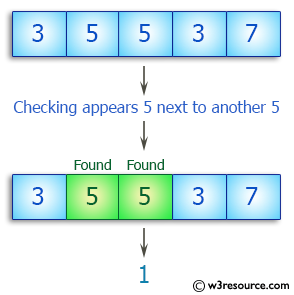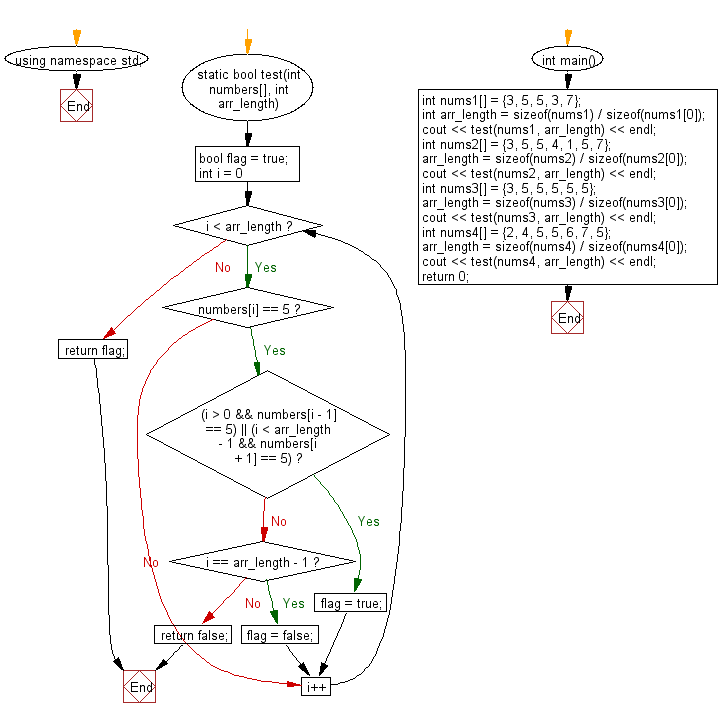﻿ C++ : Check every 5 appears in an array is next to another 5# C++ Exercises: Check a given array of integers and return true if every 5 that appears in the given array is next to another 5

## C++ Basic Algorithm: Exercise-113 with Solution

Write a C++ program to check a given array of integers and return true if every 5 that appears in the given array is next to another 5.

Sample Solution:

C++ Code :

``````#include <iostream>
using namespace std;

static bool test(int numbers[], int arr_length)
{
bool flag = true;

for (int i = 0; i < arr_length; i++)
{
if (numbers[i] == 5)
{
if ((i > 0 && numbers[i - 1] == 5) || (i < arr_length - 1 && numbers[i + 1] == 5)) flag = true;
else if (i == arr_length - 1) flag = false;
else return false;
}
}
return flag;
}

int main()
{
int nums1[] = {3, 5, 5, 3, 7};
int arr_length = sizeof(nums1) / sizeof(nums1);
cout << test(nums1, arr_length) << endl;
int nums2[] = {3, 5, 5, 4, 1, 5, 7};
arr_length = sizeof(nums2) / sizeof(nums2);
cout << test(nums2, arr_length) << endl;
int nums3[] = {3, 5, 5, 5, 5, 5};
arr_length = sizeof(nums3) / sizeof(nums3);
cout << test(nums3, arr_length) << endl;
int nums4[] = {2, 4, 5, 5, 6, 7, 5};
arr_length = sizeof(nums4) / sizeof(nums4);
cout << test(nums4, arr_length) << endl;
return 0;
}
``````

Sample Output:

```1
0
1
0
```

Pictorial Presentation:Flowchart:C++ Code Editor:

Contribute your code and comments through Disqus.

What is the difficulty level of this exercise?

﻿